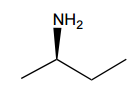Ch. 15 - Analytical Techniques: IR, NMR, Mass SpectWorksheetSee all chapters
 Ch. 1 - A Review of General Chemistry 4hrs & 47mins 0% complete Worksheet Ch. 2 - Molecular Representations 1hr & 12mins 0% complete Worksheet Ch. 3 - Acids and Bases 2hrs & 45mins 0% complete Worksheet Ch. 4 - Alkanes and Cycloalkanes 4hrs & 18mins 0% complete Worksheet Ch. 5 - Chirality 3hrs & 33mins 0% complete Worksheet Ch. 6 - Thermodynamics and Kinetics 1hr & 19mins 0% complete Worksheet Ch. 7 - Substitution Reactions 1hr & 46mins 0% complete Worksheet Ch. 8 - Elimination Reactions 2hrs & 24mins 0% complete Worksheet Ch. 9 - Alkenes and Alkynes 2hrs & 10mins 0% complete Worksheet Ch. 10 - Addition Reactions 3hrs & 33mins 0% complete Worksheet Ch. 11 - Radical Reactions 1hr & 57mins 0% complete Worksheet Ch. 12 - Alcohols, Ethers, Epoxides and Thiols 2hrs & 34mins 0% complete Worksheet Ch. 13 - Alcohols and Carbonyl Compounds 2hrs & 14mins 0% complete Worksheet Ch. 14 - Synthetic Techniques 1hr & 28mins 0% complete Worksheet Ch. 15 - Analytical Techniques: IR, NMR, Mass Spect 7hrs & 18mins 0% complete Worksheet Ch. 16 - Conjugated Systems 5hrs & 49mins 0% complete Worksheet Ch. 17 - Aromaticity 2hrs & 24mins 0% complete Worksheet Ch. 18 - Reactions of Aromatics: EAS and Beyond 4hrs & 31mins 0% complete Worksheet Ch. 19 - Aldehydes and Ketones: Nucleophilic Addition 4hrs & 54mins 0% complete Worksheet Ch. 20 - Carboxylic Acid Derivatives: NAS 2hrs & 3mins 0% complete Worksheet Ch. 21 - Enolate Chemistry: Reactions at the Alpha-Carbon 1hr & 59mins 0% complete Worksheet Ch. 22 - Condensation Chemistry 2hrs & 13mins 0% complete Worksheet Ch. 23 - Amines 1hr & 43mins 0% complete Worksheet Ch. 24 - Carbohydrates 5hrs & 56mins 0% complete Worksheet Ch. 25 - Phenols 15mins 0% complete Worksheet Ch. 26 - Amino Acids, Peptides, and Proteins 2hrs & 54mins 0% complete Worksheet

Concept #1: General Assumption for 1H NMR Signals

Transcript

Let's discuss the first piece of information that we can derive from a proton NMR and that's the total number of signals.
On a typical proton NMR, there's going to be as many signals on the spectrum as there are unique non-equivalent types of protons. For that, we need to understand what's an equivalent or non-equivalent proton. Well, an equivalent proton is going to be a proton that has the same perspective on the molecule as another proton. If two protons are in pretty much the same place on a molecule, like for example, let's say the three protons that are attached to a methyl group. A methyl group usually has three H's on it, so all three of those would be said to have the same position on the molecule, so all three of them would be what we call equivalent.
For right now, how are we going to be able to determine if something's equivalent or non-equivalent? We're just going to go with a really easy rule which is that let's go ahead and assume that hydrogen is bound to the same atom or equivalent. So like I just said, the three hydrogens on a methyl group or on a carbon would be equivalent, so that would apply to other atoms as well, not just carbon.
In general, a rule that we can go by is that any type of symmetry is going to reduce the total number of signals. This is because if you have any planes of symmetry then you're by definition going to have some protons that are the same as other protons on the other side of the molecule. Symmetry is something you have to watch for when we're using this type of information.
What we're going to do is I'm going to go ahead and do practice problem (a) as a worked example and then I'll save the other three for you guys to do on your own. Let's just go ahead and read this question. It says how many different types of protons or signals are there on each molecule. Let's look at (a).
What we notice is that (a) has obviously a bunch of hydrogens on it that aren't drawn, but it has four different atoms. What I'm wondering is how many different signals do you think this is going to have. Now notice three of them are carbon, one of them is oxygen. Is there any plane of symmetry, etcetera. Those are the things we need to be thinking about.
Well, I'm just going to tell you right now. The answer is that there are going to be four different types of hydrogens here. Let's see why. The reason is because we could just start counting from the oxygen. Let's say that the oxygen is going to be letter A so the oxygen has a hydrogen that's attached to it. And there's no other hydrogen like that, so for sure that's one type of hydrogen. No other hydrogens on that molecule look like that one.
Now we have all the rest of these hydrogens. You might have through that we could group them all together since they're all on carbon, so maybe you're thinking you have one type of hydrogen with the O and the second type is on the carbons. But it turns out that no, there are actually more separated than that because, for example, the hydrogens that are attached to this carbon are closer to the oxygen than the hydrogens attached to this carbon. That means that theoretically, the red hydrogens, the two hydrogens that are on this red carbon, are going to be a little bit more deshielded than the blue ones because they're going to be closer to something electronegative. So I would actually expect that the red ones would be a little bit more downfield because remember those words downfield and up-field.
Anyway, because of the fact those two red hydrogens are attached to the same atom, we're going to say that's the second type. The two hydrogens attached to this atom are the third type and then finally the three hydrogens on this last carbon are the fourth. So in total, we get one, two, three, four different types of protons. So not so bad.
Another thing to note is you might have been thinking maybe there was symmetry here, but really this molecule isn't symmetrical. The way that it's drawn, the oxygen is on one side and then you've got this asymmetry that goes through the whole molecule so that's why every single atom needed its own peak or its own signal.
Now go ahead with that knowledge, try to do question (b) and then I'll go ahead and solve it.

Example #1: Identifying Proton Signals

Transcript

All right guys, what was the answer for question (b). Three. Let's go ahead and check it out.
First of all, did you guys find any symmetry in this molecule? Actually yes, this is molecule with a plane of symmetry down the middle. That means that whatever conclusion I make let's say about this carbon over here, also to applies to the one across from it, meaning that if you are able to identify the amount of unique hydrogens on one side of that dotted line, the same exact thing applies to the other side, so you don't even count the ones on the other side.
For example, I noticed that this one is on a double bond, so I'm going to make this as hydrogen type A. Then I notice that these hydrogens are on an alkane, a regular sp3 hybridized carbon. That's going to be another type of hydrogen. Now I also notice that there's this carbon here. I'm wondering did you guys give that a signal or not. Actually, this carbon doesn't even count because that carbon doesn't have any hydrogens. Remember this is called proton NMR because it only responds to protons. Even though that is a unique position on the molecule, it doesn't have hydrogens so we don't count it.
Then finally we have over here, we have the hydrogens that are on that one. That's its own unique place.
We've got those three different signals. Now would we also have to draw the signals on the other side? No, because this one is also A. This one is also B. And this one is also C. That's what you do with a plane of symmetry. It means that any conclusions you have about one side, are going to be the same exact ones on the next side.
Awesome. So three different ones. Let's go ahead and move on to the next question.

Example #2: Identifying Proton Signals

Transcript

And the answer for problem C was just one, okay? So, I know some of you guys got back because it turns out, first of all, is there any symmetry? actually yes, there's actually two planes of symmetry, there's a plane of symmetry here, I'll make that the red plane and there's also plane of symmetry here, I'll make that the blue plane, okay? Now, what does it means to have a plane of symmetry again? it means that basically whatever you figure out for one side of the mirror applies to the other, okay? In this case since I have two overlapping planes of symmetry that means all I need to do is figure out the number of unique positions in one quadrant and that will apply to all the other three, okay? So, all I need to figure out is how many different positions do I have in one quadrant, okay? And, what we notice is in that one quadrant we have only two different positions, we have the red position and we have the blue position, okay? So, my question is, which of these get signals, okay? Well, red. Notice red actually already has four bonds. So, red isn't going to count, red isn't going to get a signal. Now, blue is going to get a signal but notice that this blue is the same as this one, it's the same, oops, trying to use blue here, the same as this one this one this one, it's also the same as this, okay? Those are all the same, so the answers that you're just going to get one. So, I'm just going to put the letter A because there's nothing else, okay? Awesome next question.

Example #3: Identifying Proton Signals

Transcript

So, what was the answer that you got for d? Well, this was actually kind of a high number, I want to start off by just talking about symmetry, were you able to find any symmetry on this molecule? if you did you're kind of like deluding yourself because it actually isn't, this is an asymmetrical molecule.

Now, it would actually be easy to turn it into a symmetrical molecule if you didn't have this part, let's say this ethyl group didn't exist, that would be symmetrical because now we would have a dotted line down the middle and now, anything that I determined for one half applies to the other, but that's not the molecule I gave you, I gave you a molecule that the two alkanes are symmetrical but then one side has an ethyl on the other side doesn't, that means there's no symmetry, that literally means that every single atom needs its own signals. So, I'm going to get atom A, signal B, signal C, signal D, signal E, signal F, signal G, and signal H, okay? So, all of those are going to get their own unique signal, it's eight signals. Now, you might be wondering guys, I know a few of you guys are wondering, Johnny why did you give A and F asked different signals, they look just the same to me, okay? Why would you give them different signals? Well, notice, F is closer to the ethyl group and A is further from the ethyl group, so that means there isn't perfect symmetry here, right? If the ethyl group didn't exist then A and F would have been the same thing but since that ethyl group is there there's no symmetry, that means that A and A are their own unique Peaks, okay? So, hopefully that makes sense so far, let's move on to the next part.

Practice: How many types of electrically unique protons (peaks) are there in the following molecule?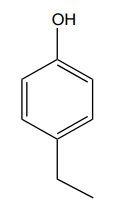Practice: How many types of electrically unique protons (peaks) are there in the following molecule?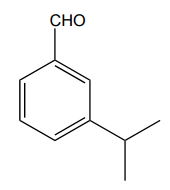Practice: How many types of electrically unique protons (peaks) are there in the following molecule?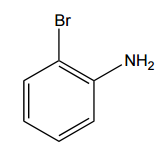Practice: How many types of electrically unique protons (peaks) are there in the following molecule?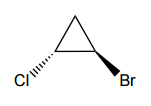Practice: How many types of electrically unique protons (peaks) are there in the following molecule?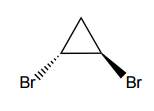Practice: How many types of electrically unique protons (peaks) are there in the following molecule?